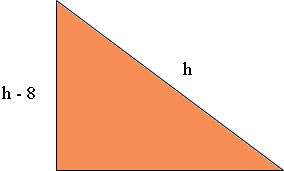SEARCH HOMEMath Central Quandaries & QueriesSubject: Triangles Name: Tayler Who are you: Student One leg of a right triangle is 8 cm. less than the hypotenuse while the other leg is 1 cm. less than the hypotenuse. Find the length of the hypotenuse.Tayler,

In my diagram I let the length of the hypotenuse be h cm. Since one of the sides has length 8 cm less than the length of the hypotenuse it has length h - 8 cm.In a similar way find the length of the third side. Now use Pythagoras' theorem to find a quadratic equation in h which you can solve to find the length of the hypotenuse.

PennyMath Central is supported by the University of Regina and The Pacific Institute for the Mathematical Sciences.